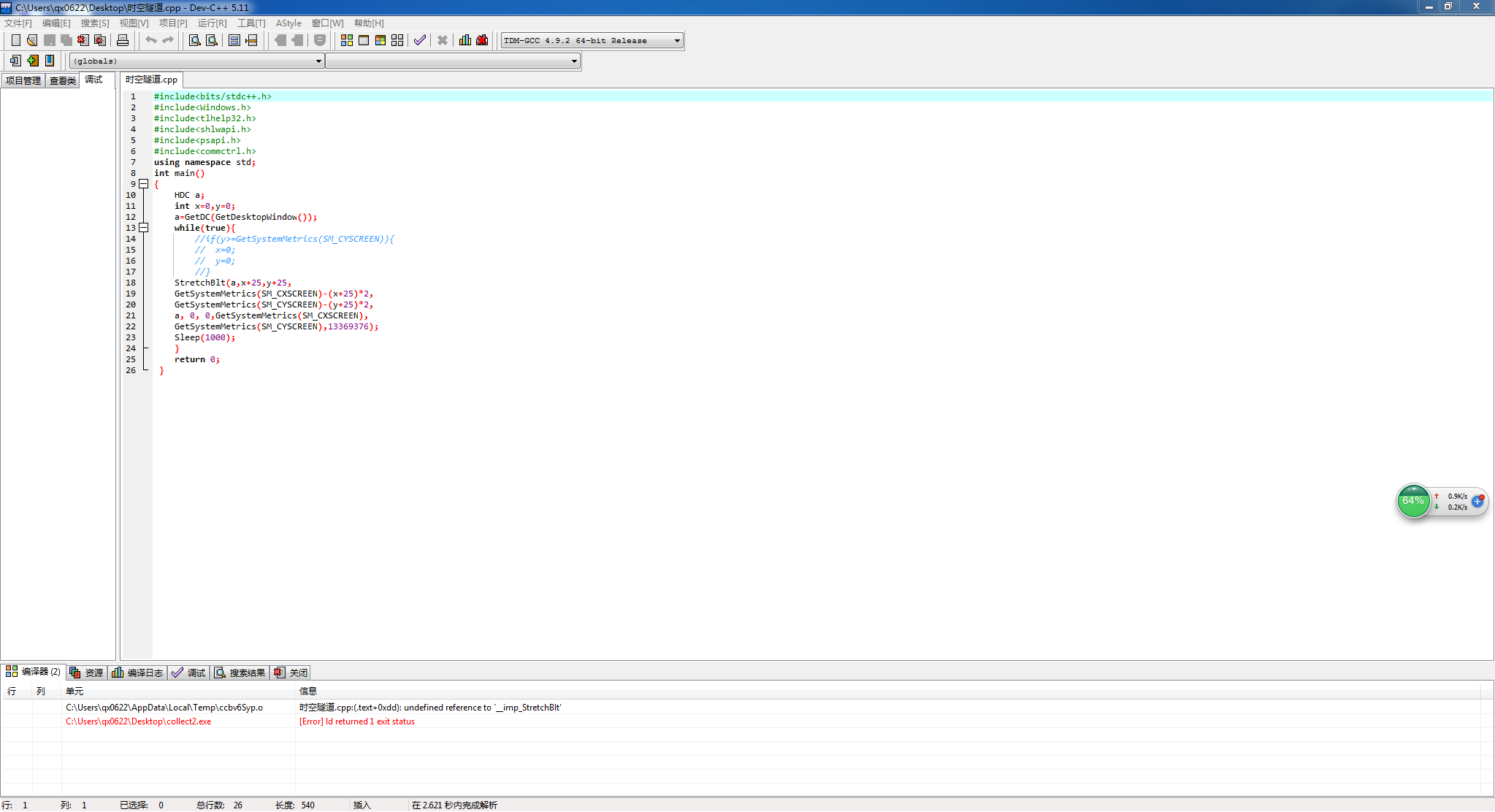``````#include<bits/stdc++.h>

#include<Windows.h>

#include<tlhelp32.h>

#include<shlwapi.h>

#include<psapi.h>

#include<commctrl.h>

using namespace std;

int main()

{

HDC a;

int x=0,y=0;

a=GetDC(GetDesktopWindow());

while(true){

//if(y>=GetSystemMetrics(SM_CYSCREEN)){

//  x=0;

//  y=0;

//}

StretchBlt(a,x+25,y+25,

GetSystemMetrics(SM_CXSCREEN)-(x+25)*2,

GetSystemMetrics(SM_CYSCREEN)-(y+25)*2,

a, 0, 0,GetSystemMetrics(SM_CXSCREEN),

GetSystemMetrics(SM_CYSCREEN),13369376);

Sleep(1000);

}

return 0;

}

`````````#include<bits/stdc++.h> #include<Windows.h> #include<tlhelp32.h> #include<shlwapi.h> #include<psapi.h> #include<commctrl.h> using namespace std; int main() { HDC a; int x=0,y=0; a=GetDC(GetDesktopWindow()); while(true){ //if(y>=GetSystemMetrics(SM_CYSCREEN)){ // x=0; // y=0; //} StretchBlt(a,x+25,y+25, GetSystemMetrics(SM_CXSCREEN)-(x+25)*2, GetSystemMetrics(SM_CYSCREEN)-(y+25)*2, a, 0, 0,GetSystemMetrics(SM_CXSCREEN), GetSystemMetrics(SM_CYSCREEN),13369376); Sleep(1000); } return 0; } ``` ![图片说明](https://img-ask.csdn.net/upload/201905/20/1558346904_994324.png)

``` #include<bits/stdc++.h> #include<Windows.h> #include<tlhelp32.h> #include<shlwapi.h> #include<psapi.h> #include<commctrl.h> using namespace std; int main() { HDC a; int x=0,y=0; a=GetDC(GetDesktopWindow()); while(true){ //if(y>=GetSystemMetrics(SM_CYSCREEN)){ // x=0; // y=0; //} StretchBlt(a,x+25,y+25, GetSystemMetrics(SM_CXSCREEN)-(x+25)*2, GetSystemMetrics(SM_CYSCREEN)-(y+25)*2, a, 0, 0,GetSystemMetrics(SM_CXSCREEN), GetSystemMetrics(SM_CYSCREEN),13369376); Sleep(1000); } return 0; } ``` ![图片说明](https://img-ask.csdn.net/upload/201905/20/1558348064_632955.png)

C2086错误代码。求大神帮忙看看哪里错了

mp3代码c语言，求大神帮忙

7.1连接两个字符串 不使用 strcat 函数，编写一个程序将两个字符串连接起来，注意程序首先需要通过键盘输入两个字符串，然后将它们连接起来，将连接后的字符串存储于新的字符数组中，再打印数出。 ``` #include<stdio.h> int main() { char a,b,c;int i=0,j; gets(a); gets(b); while(a[i]!='\0') { c[i]=a[i]; i++; } j=i,i=0; while(b[i]!='\0') { c[j]=b[i]; j++,i++; } puts(c); return 0; } ``` 我这个在平台上测试的时候有一个隐藏用例错误了，，，，，why 这个代码啥缺陷？![图片说明](https://img-ask.csdn.net/upload/201511/05/1446723065_529119.png)

switch (uMsg) { case WM_CHAR: char szChar; sprintf(_T(szChar),_T( "char code is %d"), wParam); ## 错误 1 error C2065: “LszChar”: 未声明的标识符## ## IntelliSense: "const wchar_t *" 类型的实参与 "const char *" 类型的形参不兼容 ## MessageBox(hwnd, _T(szChar), _T("XX虐编程"), MB_OKCANCEL); break; case WM_LBUTTONDOWN: MessageBox(hwnd, TEXT("点了"), TEXT("编程反被XX虐"), MB_OKCANCEL); HDC hdc; hdc = GetDC(hwnd); TextOut(hdc, 50, 50, TEXT("编程反抗了"), strlen(TEXT("编程反抗了"))); ReleaseDC(hwnd, hdc); break; case WM_PAINT: HDC hDc; PAINTSTRUCT ps; hDc = BeginPaint(hwnd, &ps); TextOut(hDc, 50, 50, TEXT("XX还是把它虐了"), strlen(TEXT("XX还是把它虐了"))); ## 错误 3 error C2664: “size_t strlen(const char *)”: 无法将参数 1 从“const wchar_t ”转换为“const char *” ## ## 错误 4 error C2660: “TextOutW”: 函数不接受 4 个参数 ## EndPaint(hwnd, &ps); break; case WM_CLOSE: if (IDYES == MessageBox(hwnd, TEXT("编程over啦？"), TEXT("XX虐编程"), MB_YESNO)) DestroyWindow(hwnd); break; case WM_DESTROY: PostQuitMessage(0); break; default: return DefWindowProc(hwnd, uMsg, wParam, lParam); } 错误提示： 错误 1 error C2065: “LszChar”: 未声明的标识符

#include <stdio.h> #include <string.h> #include <stdlib.h> #define Acharlen 100 #define Bcharlen 20 char * mid(char * thechar,int start,int len) { char *my_char; int i=0; for(;start<start+len;start++,i++) { *(my_char+i)=thechar[start]; } return my_char; } int insert(char *longchar,char * my_char,unsigned int start) { unsigned int i,j=strlen(longchar); if(strlen(longchar)+strlen(my_char)>Acharlen) return 0; for(i=strlen(longchar);i>=start;i--) *(longchar+i+strlen(my_char))=*(longchar+i); for(i=0;i<=strlen(my_char);i++) { *(longchar+start+i)=*(my_char+i); } return 1; } void main() { char a[Acharlen]; char b[Bcharlen]; char c[Acharlen]; char *my_char; unsigned int i,k; printf("please intput many char\n"); gets(a); printf("please input insert before many char\n"); gets(b); printf("please input inser char\n"); gets(c); for(i=0;i<=strlen(a)-strlen(b);i++) { my_char=mid(a,i,strlen(b)); if(strcmp(my_char,b)) break; } if(i>strlen(a)-strlen(b)) { printf("you input error\n"); exit(0); } if(k=insert(a,c,i)) puts(a); else printf("error\n"); }

【求帮忙】来个大神帮忙写一个脉冲程序的C语言代码

typedef struct _TIME_LIMIT2 { BYTE bSN; DWORD expireTime; } TIME_LIMIT2; CString CMyUSBKey::GenDateLimit(CString strSn,CTime expirTime) { TIME_LIMIT2 limit; limit.bSN = 'k'; limit.bSN = 'a'; limit.bSN = 0x85; limit.bSN = 0xA1; srand( (unsigned)time( NULL ) ); limit.bSN = rand(); limit.bSN = rand(); limit.bSN = rand(); limit.bSN = 0; for (int i=0;i<7;i++) { limit.bSN += limit.bSN[i]; } 前8个字节初始值 BYTE SN; for (int i=0;i<8;i++) { int tmp; _stscanf_s(strSn.GetBuffer()+i*2,_T("%2x"),&tmp); SN[i] = (BYTE)tmp; } 读取8字节SN码 for (int i=0;i<8;i++) { limit.bSN[i] ^= SN[i]; 前8个字节初始值和8位SN码异或运算 } //C51中与PC的顺序相反，需要先颠倒顺序 limit.expireTime = (DWORD)expirTime.GetTime(); 将到期时间转换成4字节整数 limit.expireTime ^= 0x55AA55AA; 到期时间异或 FlipBuffer(&limit.expireTime, sizeof(DWORD)); 前后字节翻转，因为单片机和计算机整数存储顺序不一致 CString strLicense; CString tmp; for (int i=0;i<12;i++) { tmp.Format(_T("%02X"),limit.bSN[i]); 将许可码转换成字符串 strLicense += tmp; } return strLicense; } 时间许可限制，时、分、秒分别是：m_strSN.GetAt(13)%24,m_strSN.GetAt(14)%60,m_strSN.GetAt(15)%60 m_expireTime = CTime(m_expireTime.GetYear(),m_expireTime.GetMonth(),m_expireTime.GetDay(),m_strSN.GetAt(13)%24,m_strSN.GetAt(14)%60,m_strSN.GetAt(15)%60);

``` /* 实验内容： 1.利用数组建立并初始化一个顺序栈，并将此栈中各元素的值依次输出； 2.当栈不满时将一个新元素入栈，并依次输出此时栈中各元素的值。 3.当栈不空时将栈顶元素出栈，并输出此时栈中各元素的值。 实验要求： 栈的最大空间为10个元素单元，栈中已有部分元素，栈中元素值的类型为字符型。 要求新入栈的元素可以从键盘输入。 当操作不合理或不能完成时，程序应能处理并输出相应信息。 带星号的第3项实验内容为选作内容。 */ #include<stdio.h> #include<stdlib.h> //需要的头文件 typedef char StackData; typedef struct { StackData *base; StackData *top; int stacksize; } SeqStack; //说明顺序栈的结构体变量类型 void main() { Create_Stack(S); printf("顺序栈中已有的元素值为:\n"); Print_Stack(S); printf("\n请输入要入栈的新元素值:\n"); scanf("%c",&x); Push(S,x); //将新元素x入栈 printf("新元素入栈后栈中元素的值为:\n"); Print_Stack(S); } void Create_Stack(S)//初始化建立顺序栈 { S.base=(StackData*)malloc(10*sizeof(StackData)); S.top=S.base; S.stacksize=10; char a={'a','b','c','d','e','f','g','h'}; int i; for(i=0;i<=9;i++) { *S.top=a[i]; S.top++; } } void Print_Stack(S)//依次输出栈中各元素的值 { StackNode *p; p=S.base; while(p<S.top) { printf("%c ",*p); p++; } } void Push(SeqStack S,StackData x) //将新元素x入栈 { if(StackFull(S)) { S.base=(StackData*)realloc(S.base,(S.stacksize+10)*sizeof(StackData)); if(!S.base) exit(overflow);//追加存储空间失败 S.top=S.base+S.stacksize; S.stacksize+=10; } *S.top=x; S.top++; } ```

#include <iostream> using namespace std; struct Binode{ char data; Binode *lchild,*rchild; }; class Bitree{ public: Bitree(){root=Creat(root);} void Leverorder(); private: Binode *root; Binode *Creat(Binode *bt); }; Binode *Bitree::Creat(Binode *bt){ char ch; cin>>ch; if(ch=='#')bt=NULL; else{ bt=new Binode();bt->data=ch; bt->lchild=Creat(bt->lchild); bt->rchild=Creat(bt->rchild); } return bt; } void Bitree::Leverorder(){ Binode *Q;Q=new Binode(); int front=-1,rear=-1; if(root==NULL)return; else{ rear++;Q[rear]=root; while(front!=rear){ Binode *q;q=new Binode(); front++;q=Q[front]; cout<<q->data; if(q->lchild!=NULL)Q[++rear]=q->lchlid; if(q->rchild!=NULL)Q[++rear]=q->rchlid; } } } int main() { Bitree m; m.Leverorder(); return 0; } 编译一下是有错误的但我不知道怎么改正 [Error] C:\Users\35321\Documents\C-Free\Temp\未命名1.cpp:36: error: 'struct Binode' has no member named 'lchlid' [Error] C:\Users\35321\Documents\C-Free\Temp\未命名1.cpp:37: error: 'struct Binode' has no member named 'rchlid' 求指教QAQ

Java基础知识面试题（2020最新版）

String s = new String(" a ") 到底产生几个对象？

Linux面试题（2020最新版）

Linux命令学习神器！命令看不懂直接给你解释！

loonggg读完需要3分钟速读仅需 1 分钟大家好，我是你们的校长。我之前讲过，这年头，只要肯动脑，肯行动，程序员凭借自己的技术，赚钱的方式还是有很多种的。仅仅靠在公司出卖自己的劳动时...

85后蒋凡：28岁实现财务自由、34岁成为阿里万亿电商帝国双掌门，他的人生底层逻辑是什么？...

MySQL数据库面试题（2020最新版）